# Calculus

You are currently browsing articles tagged Calculus.

## Calculus Review Videos

Here are some quick review videos for MAE 113.

Derivative Rules

Applications of the Derivative

Solutions

## Assignment 8

I have posted assignment 8 here. The solutions for Section 11.3 are here. The solutions for Section 11.4 are here.

Tags: , ,

## Integrals, integrals, integrals

##### List of indefinite integrals
•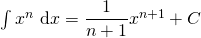(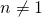)
•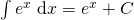and the related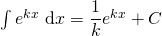()
•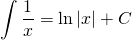•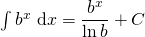()

Any of the above forumale can be verified by differentiating the right hand side.  For example() because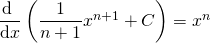.  This gives us a way to check our answers.

We can apply the rules from the list of indefinite integrals above to more complicated functions.

##### Two Useful Properties of Integrals
1.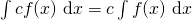The integral of a constant times a function is equal to the constant times the integral of the function.  Basically, this means that we can pull the constant out in front of the integral.  This is similar to our constant multiple rule with derivatives.

2.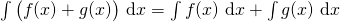The integral of a sum of two functions is equal to the integral of the first function plus the integral of the second function. Basically, this means that the integral of the sum of two functions can be broken into two separate integrals for each function.

Examples.

1.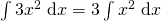by property 1.
2.by property 2.
##### Examples Illustrating the Use of the Two Properties and the List of Indefinite Integrals
1. Find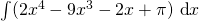.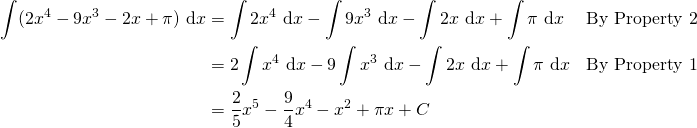2. Evaluate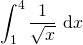.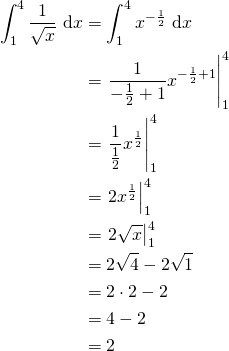3. Find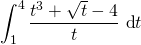.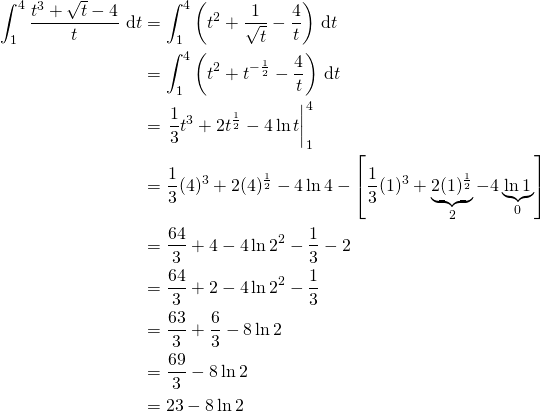Tags: , ,

## Assignment 6 & 7

Assignment 6 has been handed out. You can find a copy of it here.

Also, assignment 7 has been handed out. You can find a copy of it here.

Each of these assignments refer to the handouts that I gave in class. If you do not have the handouts, please come see me.

Tags: , ,

## Some homework questions on logarithms

Do the questions from the large booklet that I handed out to you after the test.

You should have done Exercise 1 (all questions) already as instructed at the end of the test.

Exercise 2 (p. 352-3)

1. b, d, f, h
2. b, d, f, h
3. a, c, e, g, j
4. a, c, e, g, h

Exercise 3 (p. 354)

1. a, b, d, g, h, i, j
2. a, e, f
3. b, c, d, e, f

## Assignment 5

Assignment 5 is posted here. Solutions were handed out in class.

Tags: , ,

## Test 1

The first test of MAE 113 will be on 13 February 2018.

No calculators, notes, or textbooks will be allowed.

You are responsible for all material

• covered in class lectures
• Assignments 1, 2, 3, and 4
• will be asked to prove one of the following differentiation rules:

Tips:

1. Review your two marked quizzes and identify your mistakes. You do not want to make these same mistakes again on the test.
2. Practice. You should have completed all the assignments to feel confident in abilities.
3. Drop-In Math Help Centre. It is open from 7 pm to 10 pm in G327. Tutors are available to help you. Take advantage of this opportunity!

Tags: ,

## The Birth of Calculus

This is a BBC video from 1986 on the development of calculus. For those that are interested in history, the presenter is looking at the original notes of Leibniz while he was developing calculus. He formalized the rules of differentiation within a 3 week timeframe.

## A second (more elegant) proof of the Quotient Rule

The quotient rule proof can be quite tedious if we are only allowed to use the definition of the derivative. In this post, this presents an alternative way of proving the quotient rule if we are allowed to use the product rule.

The Quotient Rule: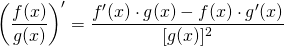Tags: , ,

## A proof of the quotient rule

The quotient rule proof can be quite tedious if we are only allowed to use the definition of the derivative.

The Quotient Rule:Tags: , ,

« Older entries